• Matlab数据降维工具箱，包括几乎所有的数据降维方法：PCA、LDA、ICA、MDS、Isomap、LandmarkIsomap、LLE、LLC、Laplacian、HessianLLE、LTSA、DiffusionMaps、KernelPCA、KernelLDA、SNE、NPE、LPP、SPE、LLTSA、...
• 囊括了几乎所有的数据降维算法，Matlab数据降维drtoolbox工具箱
• Matlab数据降维工具箱 Matlab Toolbox for Dimensionality Reduction Matlab数据降维工具箱，包括几乎所有的数据降维方法：PCA、LDA、ICA、MDS、Isomap、LandmarkIsomap、LLE、LLC、Laplacian、HessianLLE、LTSA、...
• Matlab数据降维工具箱 Matlab Toolbox for Dimensionality Reduction Matlab数据降维工具箱，包括几乎所有的数据降维方法：PCA、LDA、ICA、MDS、Isomap、LandmarkIsomap、LLE、LLC、Laplacian、HessianLLE、LTSA、...
• 数据类型MATLAB提供了多种数据类型，以供用户在不同的情况下使用。用户可以建立浮点型或者整型矩阵和数组、字符和字符串、逻辑true或者false、函数句柄、结构(Structures)数组、元胞(cell)数组、Map容器、日期和时间...


数据类型
MATLAB提供了多种数据类型，以供用户在不同的情况下使用。用户可以建立浮点型或者整型矩阵和数组、字符和字符串、逻辑true或者false、函数句柄、结构(Structures)数组、元胞(cell)数组、Map容器、日期和时间、分类数组(Categorical)、表格、时间序列等。
在MATLAB中任何一种数据类型都是以矩阵或者数组的形式来表示。这里说的矩阵或者数组，是指最小的00或N维任何尺寸的矩阵或者数组。
1.数值型
MATLAB中的数值型包括有符号和无符号的整数、单精度和双精度浮点数。默认情况下，MATLAB存储数据使用的是双精度浮点数。用户不可以更改默认的数据类型和精度，但是可以选择用非默认的整数或者单精度来储存矩阵或者数组。整数和单精度数组比双精度能节省更多的内存空间，灵活运用可以更高效地利用内存。
所有的数值型数组都支持基本的数组操作，比如说数组的重构、使用数学运算符等。
数值型数组或矩阵比较简单，因篇幅有限这里不再赘述。
2.逻辑型
逻辑型的数据是我们经常使用到的数据类型之一。本节介绍MATLAB中的逻辑型数据的使用方法。
2.1逻辑型简介
所谓逻辑数据类型，就是仅具有“TRUE”和“FALSE”两个数值的一种数据类型。一般来说，逻辑真用1表示，逻辑假用0表示。在MATLAB中，参与逻辑运算或者关系运算的并不一定必须是逻辑型的数据，任何数值都可以参与逻辑运算。在逻辑运算中，MATLAB将所有的非零值作为逻辑真来进行计算，而将零值作为逻辑假来计算。
和一般的数值型类似，逻辑类型的数据可以通过数值型转换，或者使用创建函数生成。
创建逻辑类型矩阵或者数组的函数主要有以下3个。
(1)logical函数。可将任意类型的数组转换成逻辑类型。其中非零元素为真，零元素为假。
(2)true函数。产生全部元素为逻辑真的数组。
(3)false函数。产生全部元素为逻辑假的数组。
【例3-1】  利用函数建立逻辑类型数组示例。
>> rand('state',0)
>> a=rand(4,3)                       % 产生测试矩阵
a =
0.9501    0.8913    0.8214
0.2311    0.7621    0.4447
0.6068    0.4565    0.6154
0.4860    0.0185    0.7919
>> a(a<0.6)=0                       % 产生测试矩阵
a =
0.9501    0.8913   0.8214
0    0.7621         0
0.6068         0    0.6154
0         0    0.7919
>> b=logical(a)                    %  计算逻辑型矩阵b
b =
1     1    1
0     1    0
1     0    1
0     0    1
>> c=true(size(a))                  %  生成全为true的矩阵
c =
1     1    1
1     1    1
1     1    1
1     1    1
>> d=false([size(a),2])               %  生成全为false的矩阵
d(:,:,1) =
0     0    0
0     0    0
0     0    0
0     0    0
d(:,:,2) =
0     0    0
0     0    0
0     0    0
0     0    0
>> whos                              %  查看现有的变量与数据类型
Name      Size             Bytes  Class     Attributes
a         4x3                 96  double
b         4x3                 12  logical
c         4x3                 12  logical
d         4x3x2               24  logical
由最后对结果的比较可以看出，逻辑类型的数组每一个元素仅占用一个字节的内存空间，所以矩阵b尽管和矩阵a的尺寸一样，但是在内存的占用上却有相当大的差距，并且属于不同的数据类型，这会影响计算的效率与数据的处理方式，因为逻辑型的数据在使用二进制进行计算时速度要快的多。
2.2 返回逻辑结果的函数
表3-1中所列的MATLAB操作将会返回逻辑型的true或者false。
需要说明的是：参与逻辑运算的数组不一定必须是逻辑类型的变量或常数，也可以是其他类型的数据，但是运算的结果一定是逻辑类型的数据。
表3-1        逻辑运算
运算符或函数说    明运算符或函数说    明&&具有短路作用的逻辑“与”操作，仅能处理标量==关系操作符，等于||具有短路作用的逻辑“或”操作，仅能处理标量~= 关系操作符，不等于&元素“与”操作关系操作符，小于|元素“或”操作> 关系操作符，大于~逻辑“非”操作<=关系操作符，小于等于xor逻辑“异或”操作>=关系操作符，大于等于any当向量中的元素有非零元素时，返回真所有以is开头的函数，cellfun测试操作all当向量中的元素都是非零元素时，返回真strcmp, strncmp, strcmpi, strncmpi字符串比较函数
所谓具有短路作用是指：在进行&&或||运算时，若参与运算的变量有多个，例如a&&b&&c&&d，若a、b、c、d等4个变量中的a为false，则后面的3个都不再被处理，运算结束，并返回运算结果false。
关系运算操作符可以适用于各种数据类型的变量或者常数，运算的结果是逻辑类型的数据。标量也可以和矩阵或者数组进行比较，比较的时候将自动扩展标量，返回的结果是和数组同维的逻辑类型数组。如果进行比较的是两个数组，则数组必须是同维的，且每一维的尺寸也必须一致。
【例3-2】  逻辑与、或、非使用示例。
>> a=[1 2 3;4 5 6];
>> b=[1 0 0;0 -2 1];
>> A=a&b                %  逻辑“与”
A =
1     0    0
0     1    1
>> B=a|b                %  逻辑“或”
B =
1     1    1
1     1    1
>> C=~b                 %  逻辑“非”
C =
0     1    1
1     0    0
【例3-3】  函数any 和all的使用示例。
>> a=[1 1 0; 1 0 0;1 0 1]
a =
1     1    0
1     0    0
1    0     1
>> A=all(a)                %  每列元素均为非零时返回真
A =
1     0    0
>> B=any(a)                %  每列元素存在非零时返回真
B =
1     1    1
本例首先创建数组a=[1 1 0; 1 0 0;1 0 1]，因为a的第1列均为1，所以all命令返回了1；而其他列含有0，所以返回了0，如结果中A显示的那样。any函数在数组一列中含有非0元素时就会返回逻辑1，所以B中的元素全部为1。
【例3-4】  isstrprop函数使用示例。isstrprop函数可以用来判断一个字符串中的各字符是否属于某一类别。
>> A = isstrprop('abc123def', 'alpha')
A =
1     1    1     0     0    0     1     1     1
本例中，'alpha'参数的作用就是判断输入字符串abc123def中哪些元素是字母。是字母的字符相对应地返回逻辑值true，也就是1。而数字所对应返回的是false，也就是0。
【例3-5】  关系运算示例。
>> a=[0 -1  2];
>> b=[-3 1  2];
>> a对应元素比较大小
ans =
0     1    0
>> a>b                                %  对应元素比较大小
ans =
1     0    0
>> a<=b                                %  对应元素比较大小
ans =
0     1    1
>> a>=b                                %  对应元素比较大小
ans =
1     0    1
>> a==b                                %  对应元素比较相等
ans =
0     0    1
>> a~=b                                %  对应元素比较不相等
ans =
1     1    0
2.3 运算符的优先级
在MATLAB语言中，可以自由组合运算符，组成更为复杂的运算表达式。需要注意的是：MATLAB语言中的运算符和其他的高级编程语言一样，具有优先级问题。对运算优先级的掌握，可以使我们正确地完成复杂的运算。下面将MATLAB语言的运算符和计算优先级，按照从高到低的顺序进行排列。
(1)括号()。
(2)数组的转秩(.’)，数组幂(.^)，复转秩(’)，矩阵幂(^)。
(3)代数正(+)，代数负(-)，逻辑非(~)。
(4)数组乘法(.*)，数组除法(./)，数组除(./)，矩阵乘法(*)，矩阵右除(/)，矩阵左除(\)。
(5)加法(+)，减法(-)。
(6)冒号运算符(:)。
(7)小于(<)，小于等于(<=)，大于(>)，大于等于(>=)，等于(==)，不等于(~=)。
(8)元素与(&)。
(9)元素或(|)。
(10)短路逻辑与(&&)。
(11)短路逻辑或(||)。
如果同一级别的运算符出现在一个表达式中，则按照运算符在表达式中出现的次序，由左到右排列。在具体的程序编写过程中，需要牢记运算符优先级并灵活使用。展开全文• drtoolbox: Matlab Toolbox for Dimensionality Reduction是Laurens van der Maaten开发的用来数据降维的工具箱，其中包含了著名的PCA,LDA算法，流行学习算法MLE,LLE,LPP,SNE，Isomap等，和度量学习算法LMNN,MCML,...
• 常用Matlab降维软件包包括真实有效的多种降维算法： - Principal Component Analysis ('PCA') - Linear Discriminant Analysis ('LDA') - Multidimensional scaling ('MDS') - Isomap ('Isomap') - Landmark ...
• 最近在做数据降维的处理工作接触到drtoolbox工具箱，感谢：http://blog.csdn.net/xiaowei_cqu 提供的博文，我又做了些总结。 1.MATLAB drtoolbox 介绍 The Matlab Toolbox for Dimensionality Reduction ...
最近在做数据降维的处理工作接触到drtoolbox工具箱，感谢：http://blog.csdn.net/xiaowei_cqu 提供的博文，我又做了些总结。
1.MATLAB drtoolbox 介绍

The Matlab Toolbox for Dimensionality Reduction contains Matlab implementations of 38 techniques for dimensionality reduction and metric learning.
官方网站：
http://homepage.tudelft.nl/19j49/Matlab_Toolbox_for_Dimensionality_Reduction.html
ThisMatlab toolbox implements 32 techniques for dimensionality reduction. Thesetechniques are all available through the COMPUTE_MAPPING function or trhoughthe GUI. The following techniques are available:
- Principal Component Analysis ('PCA')
- Linear Discriminant Analysis ('LDA')
- Multidimensional scaling ('MDS')
- Probabilistic PCA ('ProbPCA')
- Factor analysis ('FactorAnalysis')
- Sammon mapping ('Sammon')
- Isomap ('Isomap')
- Landmark Isomap ('LandmarkIsomap')
- Locally Linear Embedding ('LLE')
- Laplacian Eigenmaps ('Laplacian')
- Hessian LLE ('HessianLLE')
- Local Tangent Space Alignment ('LTSA')
- Diffusion maps ('DiffusionMaps')
- Kernel PCA ('KernelPCA')
- Generalized Discriminant Analysis('KernelLDA')
- Stochastic Neighbor Embedding ('SNE')
- Symmetric Stochastic Neighbor Embedding('SymSNE')
- t-Distributed Stochastic Neighbor Embedding('tSNE')
- Neighborhood Preserving Embedding ('NPE')
- Linearity Preserving Projection ('LPP')
- Stochastic Proximity Embedding ('SPE')
- Linear Local Tangent Space Alignment('LLTSA')
- Conformal Eigenmaps ('CCA', implemented asan extension of LLE)
- Maximum Variance Unfolding ('MVU',implemented as an extension of LLE)
- Landmark Maximum Variance Unfolding('LandmarkMVU')
- Fast Maximum Variance Unfolding ('FastMVU')
- Locally Linear Coordination ('LLC')
- Manifold charting ('ManifoldChart')
- Coordinated Factor Analysis ('CFA')
- Gaussian Process Latent Variable Model('GPLVM')
- Autoencoders using stack-of-RBMs pretraining('AutoEncoderRBM')
- Autoencoders using evolutionary optimization('AutoEncoderEA')
Furthermore,the toolbox contains 6 techniques for intrinsic dimensionality estimation.These techniques are available through the function INTRINSIC_DIM. Thefollowing techniques are available:
- Eigenvalue-based estimation ('EigValue')
- Maximum Likelihood Estimator ('MLE')
- Estimator based on correlation dimension('CorrDim')
- Estimator based on nearest neighborevaluation ('NearNb')
- Estimator based on packing numbers('PackingNumbers')
- Estimator based on geodesic minimum spanningtree ('GMST')
Inaddition to these techniques, the toolbox contains functions for prewhiteningof data (the function PREWHITEN), exact and estimate out-of-sample extension(the functions OUT_OF_SAMPLE and OUT_OF_SAMPLE_EST), and a function thatgenerates toy datasets (the function GENERATE_DATA).
Thegraphical user interface of the toolbox is accessible through the DRGUIfunction.
2.安装
将下载好的drtoolbox工具包解压到指定目录：D:\MATLAB\R2012b\toolbox
找到' D:\MATLAB\R2012b\toolbox\local\pathdef.m'文件，打开，并把路径添加到该文件中，保存。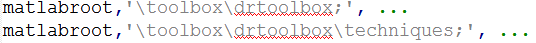运行rehash toolboxcache 命令，完成工具箱加载
>>rehashtoolboxcache
测试
>>what drtoolbox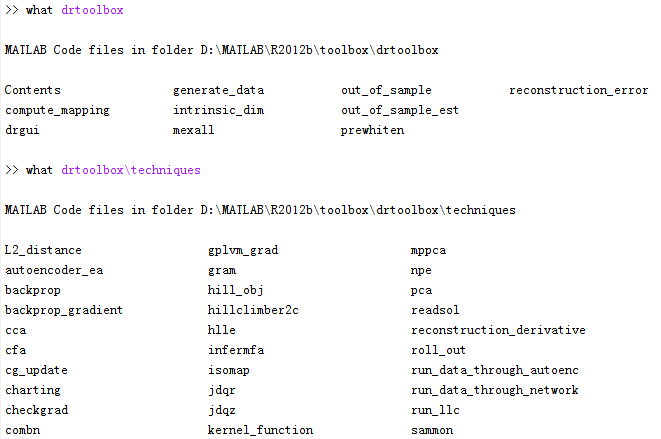3.工具箱说明
数据降维基本原理是将样本点从输入空间通过线性或非线性变换映射到一个低维空间，从而获得一个关于原数据集紧致的低维表示。
算法基本分类：
线性/非线性
线性降维是指通过降维所得到的低维数据能保持高维数据点之间的线性关系。线性降维方法主要包括PCA、LDA、LPP（LPP其实是LaplacianEigenmaps的线性表示）；非线性降维一类是基于核的，如KPCA，此处暂不讨论；另一类就是通常所说的流形学习：从高维采样数据中恢复出低维流形结构（假设数据是均匀采样于一个高维欧式空间中的低维流形），即找到高维空间中的低维流形，并求出相应的嵌入映射。非线性流形学习方法有：Isomap、LLE、LaplacianEigenmaps、LTSA、MVU
整体来说，线性方法计算块，复杂度低，但对复杂的数据降维效果较差。
监督/非监督
监督式和非监督式学习的主要区别在于数据样本是否存在类别信息。非监督降维方法的目标是在降维时使得信息的损失最小，如PCA、LPP、Isomap、LLE、LaplacianEigenmaps、LTSA、MVU；监督式降维方法的目标是最大化类别间的辨别信，如LDA。事实上，对于非监督式降维算法，都有相应的监督式或半监督式方法的研究。
全局/局部
局部方法仅考虑样品集合的局部信息，即数据点与临近点之间的关系。局部方法以LLE为代表，还包括LaplacianEigenmaps、LPP、LTSA。
全局方法不仅考虑样本几何的局部信息，和考虑样本集合的全局信息，及样本点与非临近点之间的关系。全局算法有PCA、LDA、Isomap、MVU。
由于局部方法并不考虑数据流形上相距较远的样本之间的关系，因此，局部方法无法达到“使在数据流形上相距较远的样本的特征也相距较远”的目的。
4.工具箱使用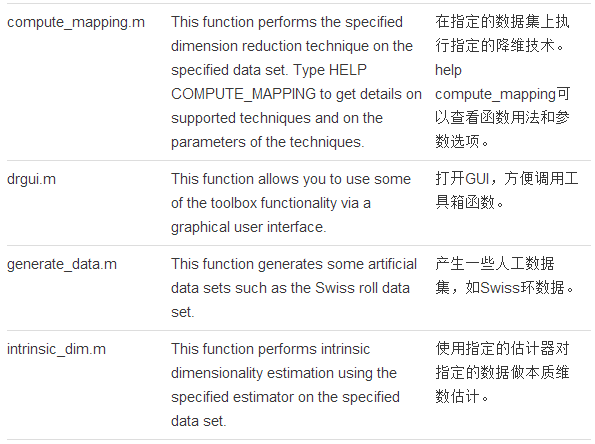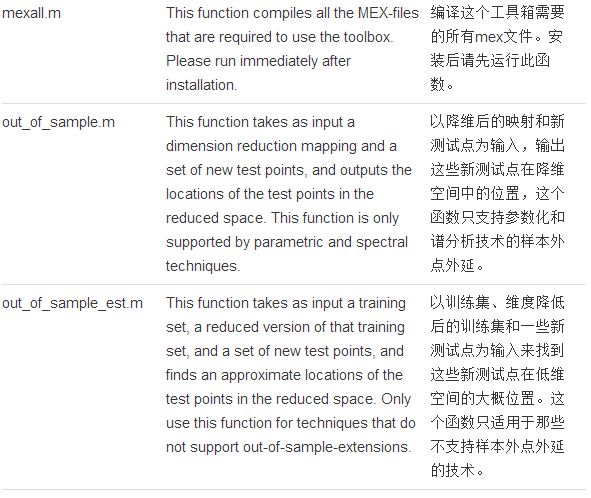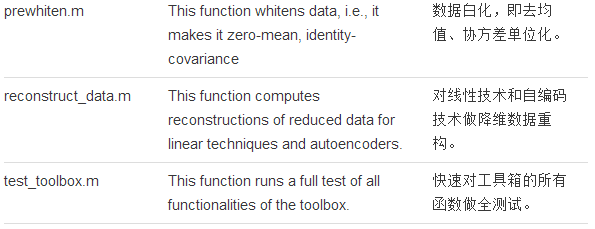使用实例：

clc
clear
close all

% 产生测试数据
[X, labels] = generate_data('helix', 2000);
figure
scatter3(X(:,1), X(:,2), X(:,3), 5, labels)
title('Original dataset')
drawnow

% 估计本质维数
no_dims = round(intrinsic_dim(X, 'MLE'));
disp(['MLE estimate of intrinsic dimensionality: ' num2str(no_dims)]);

% PCA降维
[mappedX, mapping] = compute_mapping(X, 'PCA', no_dims);
figure
scatter(mappedX(:,1), mappedX(:,2), 5, labels)
title('Result of PCA')

% Laplacian降维
[mappedX, mapping] = compute_mapping(X, 'Laplacian', no_dims, 7);
figure
scatter(mappedX(:,1), mappedX(:,2), 5, labels(mapping.conn_comp))
title('Result of Laplacian Eigenmaps')
drawnow

% Isomap降维
[mappedX, mapping] = compute_mapping(X, 'Isomap', no_dims);
figure
scatter(mappedX(:,1), mappedX(:,2), 5, labels(mapping.conn_comp))
title('Result of Isomap')
drawnow



展开全文drtoolbox
• matlab 降维工具箱及说明文档matlab 降维工具箱及说明文档
• 前言核主成分分析 (KPCA) ...KPCA通常有以下主要应用：降维特征提取去噪 故障检测由于 KPCA 的原理已经有很多文章介绍了，本文不再赘述，侧重是介绍 KPCA 的 MATLAB 实现。KPCA 建模流程KPCA 的建模流程如下：获...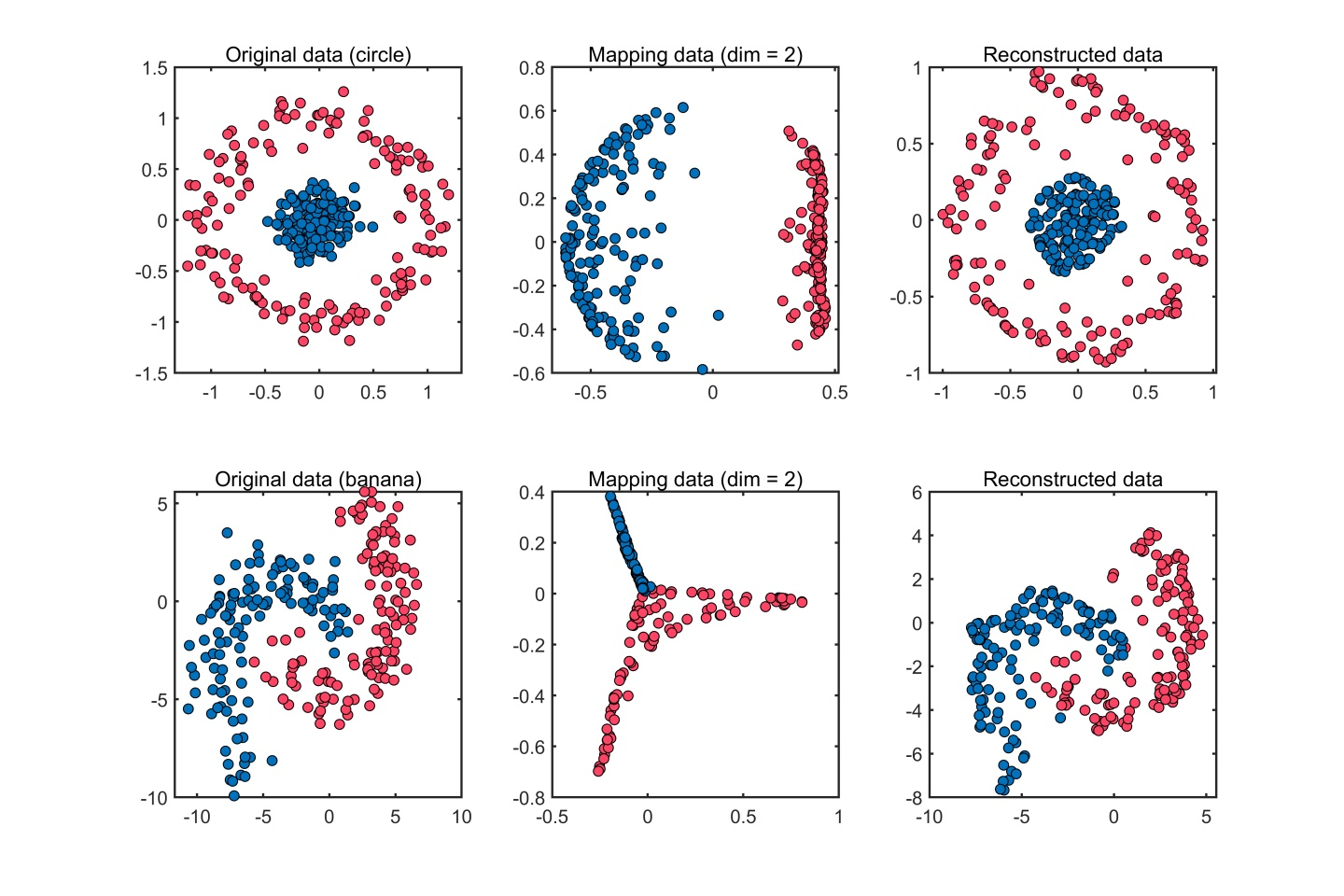前言
核主成分分析 (KPCA) 是一种非线性数据处理方法，其核心思想是通过一个非线性映射把原始空间的数据投影到高维特征空间， 然后在高维特征空间中进行基于主成分分析 (PCA) 的数据处理。KPCA通常有以下主要应用：
降维特征提取去噪 故障检测
由于 KPCA 的原理已经有很多文章介绍了，本文不再赘述，侧重是介绍 KPCA 的 MATLAB 实现。
KPCA 建模流程
KPCA 的建模流程如下：
获取训练数据计算核矩阵核矩阵中心化特征值分解特征向量的标准化处理主成分个数的选取计算非线性主成分计算 SPE 和 T2 统计量计算 SPE 和 T2 统计量的控制限
通过步骤1-7可以实现数据降维或者特征提取（即非线性主成分）。另外，基于 KPCA 的数据重构一般是通过求解近似的逆映射，把所获取的非线性主成分重构到原始空间，本文参考以下资料来实现 KPCA 数据重构的MATLAB实现：
Python 的机器学习库 Scikit-learn (sklearn)：sklearn.decomposition.KernelPCA.inverse_transformBakır G H, Weston J, Schölkopf B. Learning to find pre-images [J]. Advances in neural information processing systems, 2004, 16: 449-456.
通过步骤1-9实现故障检测模型的建立。对于测试数据，在获取 KPCA 模型的基础上，实现故障检测的流程为：
获取测试数据计算核矩阵核矩阵中心化计算测试数据的非线性主成分计算 SPE 和 T2 统计量与 SPE 和 T2 统计量的控制限进行比较若超过控制限，则判断为故障，并进行故障诊断。
对于步骤7， 通过计算故障区间的 SPE 和 T2 统计量的贡献率来实现故障诊断。本文参考以下资料来实现KPCA故障检测和故障诊断：
Lee J M, Yoo C K, Choi S W, et al. Nonlinear process monitoring using kernel principal component analysis[J]. Chemical engineering science, 2004, 59(1): 223-234. （故障检测）Deng X, Tian X. A new fault isolation method based on unified contribution plots[C]//Proceedings of the 30th Chinese Control Conference. IEEE, 2011: 4280-4285. （故障诊断）
KPCA 的 MATLAB 实现
笔者在上述资料的基础上，编写了 KPCA 的 MATLAB 实现代码，主要特点有：
易于使用的函数接口支持多种常用的核函数支持数据降维和重构支持故障检测和故障诊断
源代码已经发布在 MATLAB 中文论坛、 GitHub 和 MathWorks 的 File Exchange:

核主元分析 (KPCA)的降维、特征提取以及故障检测应用​www.ilovematlab.cnhttps://github.com/iqiukp/Kernel-Principal-Component-Analysis-KPCA​github.com

Kernel Principal Component Analysis (KPCA)​ww2.mathworks.cn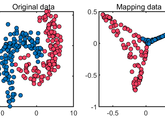下面介绍代码的组成。
核函数
类 Kernel 提供了以下6种核函数 ：
linear kernel functiongaussian kernel functionpolynomial kernel functionsigmoid kernel functionexponential kernel functionlaplacian kernel function
KPCA
KPCA的训练、测试和可视化由类 KernelPCA 、类 KernelPCAFunction 和 类 Visualization实现。
类 KernelPCA 是核心模块，方法包括：
KernelPCA：构造函数train：模型训练test：模型测试reconstruct：数据重构computeEigenvalue：特征值分解computeControlLimit：计算统计量的控制限diagnose：故障诊断computeContribution：统计量的贡献率
类 KernelPCAFunction 实现一些辅助功能，方法包括：
checkInput：初始化参数displayTrain：模型的信息输出displayTest：模型的信息输出
类 Visualization 实现实验结果的可视化，方法包括：
map：可视化降维数据（dim = 2）prediction：可视化故障检测结果reconstruct：可视化数据重构结果diagnose：可视化故障重构结果
数据降维 (特征提取) 示例 （circle 数据 和 banana 数据，高斯核函数）

clc


为了方便可视化，circle 数据 和 banana 数据都是只有2个特征的数据。其降维（特征提取）结果为：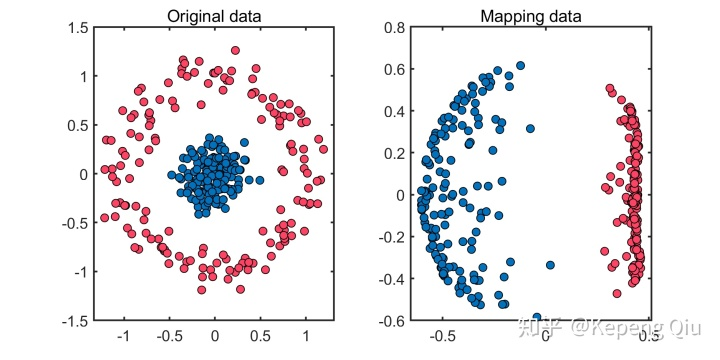circle 数据的降维 (特征提取) 结果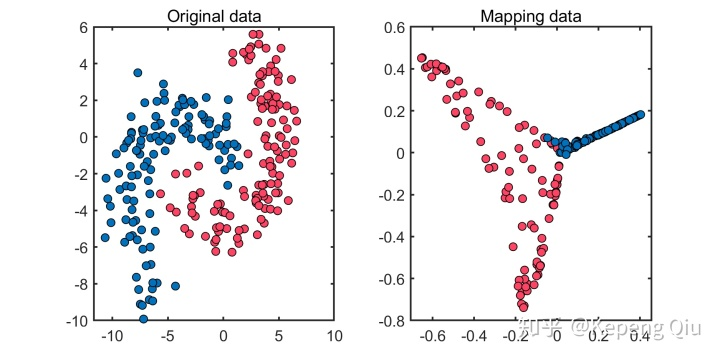banana 数据的降维 (特征提取) 结果

数据重构示例 （circle 数据 和 banana 数据，高斯核函数）

clc


数据重构结果为：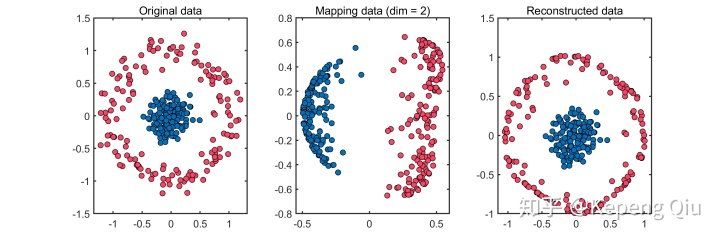circle 数据的重构结果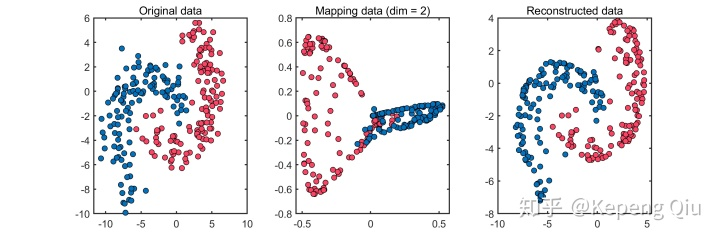banana 数据的重构结果

故障检测示例 （TE 过程数据，高斯核函数）

clc


TE过程是一个典型的工业过程，本文实验的训练数据为500*52，测试数据为960*52，数据矩阵的行为样本个数，列为特征个数。故障检测结果为：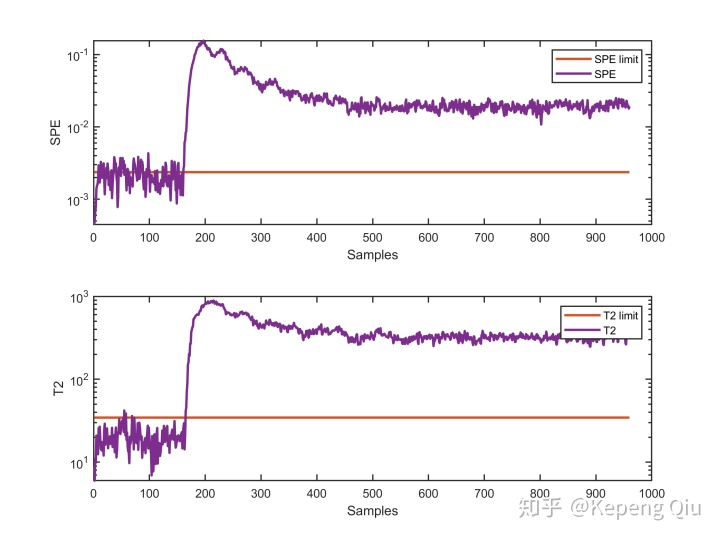故障检测结果

故障诊断示例 （TE 过程数据，高斯核函数）

clc


诊断结果：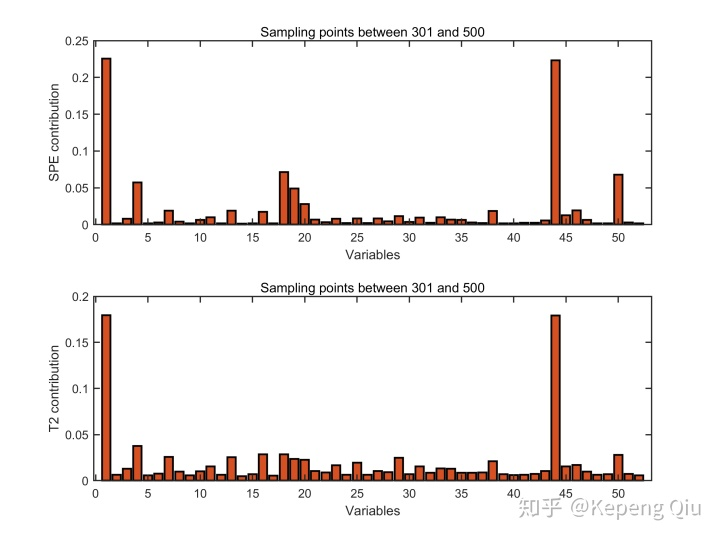故障诊断结果

注意
模型训练和测试结果存储在类 KernelKPCA 创建对象的属性 model 和 prediction 中参数说明请阅读 contents.m 文件核心模块采用 classdef ... end 的类定义，代码适用的 MATLAB 最低版本为 R2008a此代码仅供参考
小尾巴
如果这篇文章对您有所帮助，欢迎点赞、关注、收藏，同时也欢迎专注我的个人专栏，分享与交流机器学习算法和编程实践心得。

机器学习与编程实践​zhuanlan.zhihu.com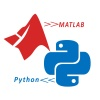参考
^Lee J M, Yoo C K, Choi S W, et al. Nonlinear process monitoring using kernel principal component analysis[J]. Chemical engineering science, 2004, 59(1): 223-234.^Bakır G H, Weston J, Schölkopf B. Learning to find pre-images [J]. Advances in neural information processing systems, 2004, 16: 449-456^https://github.com/scikit-learn/scikit-learn/blob/95d4f0841/sklearn/decomposition/_kernel_pca.py#L341^Deng X, Tian X. A new fault isolation method based on unified contribution plots[C]//Proceedings of the 30th Chinese Control Conference. IEEE, 2011: 4280-4285.

展开全文• 一 主成分分析(PAC) 遇到由多个变量决定的问题的...而在数据的处理过程中方差越大，则表示原数据波动越大，覆盖范围更广，因此选择方差最大的作为第一主成分。但是并不能有效代表原变量，因此需要找到第二主成分...
一 主成分分析(PAC)
遇到由多个变量决定的问题的时候，其变量之间存在相互关系，分析问题难度很大。因此我们可以找到具有代表性的几个变量，且变量之间不存在联系。由这几个代表性变量分析与建模解决该问题，这就是主成分分析法。
1.1 PAC基本原理
将相互关联的数据通过线性组合为互不相关的新的综合变量，在新的变量中我们要找到尽可能多的代表原变量。而在数据的处理过程中方差越大，则表示原数据波动越大，覆盖范围更广，因此选择方差最大的作为第一主成分。但是并不能有效代表原变量，因此需要找到第二主成分、第三主成分……直至有效代表原变量。并且第一主成分与第二主成分之间满足①,即第二主成分中不包含第一主成分代表的变量。
1.2 PAC步骤
（1）对原数据进行标准化处理。假设数据样本：

将数据进行如下处理：

其中，
,
（2）计算样本相关系数矩阵。假设原始数据标准化（去关联）后仍用X表示，则相关系数为：

其中：

（3）计算相关系数矩阵R的特征值和相应的特征向量：

（4）根据贡献率的大小选取k个主成分并写出表达式：

k值得选取由累计贡献率决定，累计贡献率一般为85%：

（5）计算主成分得分。

其中：

（6）依据主成分得分的数据，进一步对问题进行后续分析和建模。
1.3 MATLAB仿真练习

for i=1:M     Eg(:,i)=(Y(:,i)-mean(Y(:,i)))/std(Y(:,i));     if i==M         op=std(Y(:,i));     end end CM=corrcoef(Eg); [V,eValue]=eig(Eg); for j=1:M     DeValue(j,1)=eValue(j,j); end SV=sort(DeValue(:,1),'descend'); for k=1:M     k_value(k,1)=k;     SV(k,2)=SV(k,1)/sum(SV(:,1));     SV(k,3)=sum(SV(1:k,1))/sum(SV(:,1)); end

程序如上图所示，没有选择好的数据，因此结果并不是很适合该题。注重运算过，其结果如下
相关系数矩阵：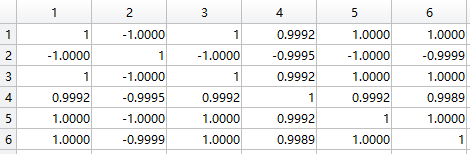特征值矩阵：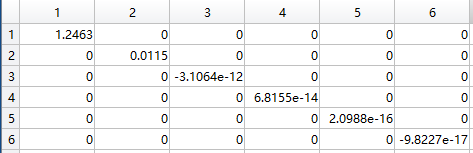特征向量矩阵：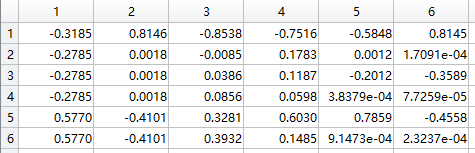特征值&贡献率&累计贡献率：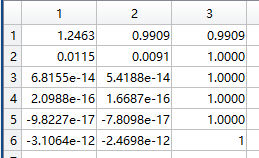可以看出计算结果在特征值得筛选上并没有效果，因此用1到6代替特征值，然后进行筛选；

s_value=sort(k_value(:,1),'descend'); for m=1:M     s_value(m,2)=s_value(m,1)/sum(s_value(:,1));     s_value(m,3)=sum(s_value(1:m,1))/sum(s_value(:,1)); end T=0.7; t=0; for n=1:M     if s_value(n,3)>=T         Com_num=n;         break;     end end

计算贡献率和累计贡献率：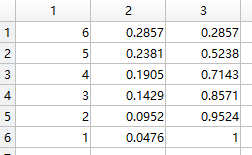将保留率设置为0.7，则可以得到结果为3，也就是所需提取的特征向量有三个，按原数据特征值向量从大到小选择：

for t_nump=1:6     if DeValue(t_nump,1)>=SV(3,1)         t=t+1;         need_n(t,1)=t_nump;     end end for t_num=1:3     need_p(:,t_num)=V(:,need_n(t_num,1)); end

选取结果：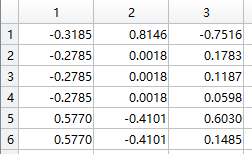然后根据公式就可以计算出主成分得分情况。

for t_num=1:3     need_p(:,t_num)=V(:,need_n(t_num,1));     need_q(:,t_num)=Eg(:,need_n(t_num,1)); end new_score=Eg*need_q; for i=1:N     total_score(i,1)=sum(new_score(i,:));     total_score(i,2)=i; end result_report=[new_score,total_score]; result_report=sortrows(result_report,-4);

得到结果如下：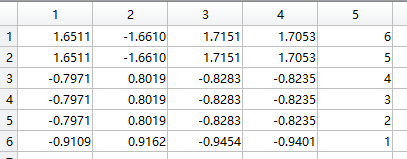①：统计学中表示协方差。
在概率论和统计学中协方差表示两个变量的总体误差，期望与协方差满足一下关系式：

当两个变量的线性无关时满足，因此当是线性无关。

卓金武，王鸿钧等，《MATLAB数学建模方法与实践》（第三版）
展开全文• 最近想做数据降维的处理工作，接触到drtoolbox工具箱 感谢：http://blog.csdn.net/xiaowei_cqu提供的博文，就文中的内容做些补充 The Matlab Toolbox for Dimensionality Reduction contains Matlab ...
• 数据降维工具箱，包括一些典型算法，例如pca，lle，mds，lda等。
• matlab drtoolbox数据降维包，包括多种降维算法...

# matlab数据降维matlab 订阅